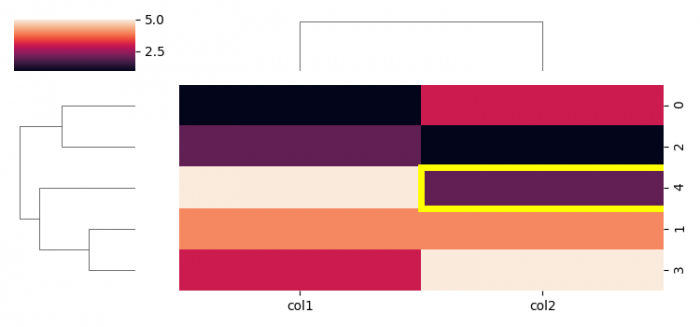# Add a custom border to certain cells in a Matplotlib / Seaborn plot

MatplotlibPythonData Visualization

To add a custom border to certain cells in a Matplotlib/Seaborn plot.

## Steps

• Set the figure size and adjust the padding between and around the subplots.
• Create a dataframe with some columns.
• Plot a matrix dataset as a hierarchically-clustered heatmap.
• Get heatmap axis as a subplot arrangement.
• To add a custom border to certain cells in Matplotlib, we can intialize a variable, border_color.
• Using custom bordder color, add a rectangle patch on the heatmap axes.
• To display the figure, use show() method.

## Example

import pandas as pd
from matplotlib import pyplot as plt
import seaborn as sns
plt.rcParams["figure.figsize"] = [7.50, 3.50]
plt.rcParams["figure.autolayout"] = True
df = pd.DataFrame({"col1": [1, 4, 2, 3, 5], "col2": [3, 4, 1, 5, 2]})
g = sns.clustermap(df, figsize=(7.50, 3.50))
ax = g.ax_heatmap
border_color = "yellow"
plt.show()